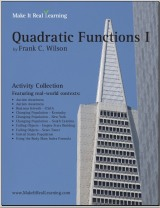August SALE! Get Math Mammoth and Make It Real Learning downloads & CDs at 25% off — right here at the MathMammoth.com website. Use coupon code V827-VMC3-HK7V at checkout. Offer valid through August 25, 2022.

-->
^
You are here: HomeMake It Real Learning → Quadratic Functions I

# Make It Real Learning Quadratic Functions I workbook46 pages
(10 activities)

Free Sample (PDF):
United States Population: Using Quadratic Models

# Introduction

The Make It Real Learning Quadratic Functions I workbook focuses on real-world situations that may be effectively modeled by quadratic functions. This book is suitable for both algebra 1 and algebra 2 students.

Whether business growth, changing population or falling objects, learners get to use mathematics in meaningful ways. Each activity integrates real world information, companies, and issues (e.g. USAA, autism, body mass index), not just "realistic" data.

There are multiple ways to use the activities of the book in a teaching environment. The activities are an excellent tool for stimulating mathematical discussions in a small group setting. Due to the challenging nature of each activity, group members are motivated to brainstorm problem solving strategies together. The interesting real world contexts motivate them to want to solve the problems. The activities may also be used for individual projects and class-wide discussions.

As a ready-resource for teachers, the workbook also includes completely worked-out solutions for each activity. To make it easier for teachers to assess student work, the solutions are included on a duplicate copy of each activity.

## Quadratic Functions I Activity Objectives

Activity TitleMathematical Objectives
Autism Awareness:
Calculate and interpret the meaning of average rates of change
Use rates of change to forecast unknown results
Use regression to find a quadratic model
Autism Awareness:
Evaluate a quadratic function model at a given value
Find the x-intercepts and vertex of a parabola from its equation
Calculate and analyze trends in rates of change
Draw a scatter plot of a data set
Use regression to find a quadratic model
Changing Population — Kentucky:
Doing Data Analysis and Modeling
Determine if a linear or quadratic function best fits a data set
Use regression to find a quadratic model
Changing Population - New York:
Explain the relationship between rates of change and concavity
Determine if a linear or quadratic function best fits a data set
Use regression to find a quadratic model
Find the vertex of a quadratic function algebraically
Changing Population — South Carolina:
Doing Data Analysis and Modeling
Determine if a linear or quadratic function best fits a data set
Use regression to find a quadratic model
Evaluate a quadratic function at a given value
Falling Objects - Empire State Building:
Use a quadratic model for the position of a falling object
Create a linear equation for the velocity of a falling object
Use the quadratic formula to find x-intercepts
Falling Objects - Sears Tower:
Use a quadratic model for the position of a falling object
Create a linear equation for the velocity of a falling object
Use the quadratic formula to find x-intercepts
United States Population:
Evaluate a quadratic function at a given value
Estimate the point of intersection of two graphs visually
Using the Body Mass Index Formula:
Solving Equations
Evaluate a multivariable function at a given point

Bundle specials!

Activity Library, Volume I. Price: \$39.99
Includes 11 Make It Real Learning activity workbooks for the price of 8! You get three workbooks for free!

Activity Library, Volume II. Price: \$39.99
Includes 11 Make It Real Learning activity workbooks for the price of 8! You get three workbooks for free!

States by the Numbers Bundle. Price: \$19.99
Inludes all 50 States by the Numbers workbooks at a whopping 87% discount!
(Normal price would be 50 × \$2.99 = \$149.50)

By purchasing any of the Make It Real Learning workbooks, permission IS granted for the teacher (or parent) to reproduce this material to be used with his/her students in a teaching situation; not for commercial resale. However, you are not permitted to share the material with another teacher.

In other words, you are permitted to make copies for the students/children you are teaching, but not for other teachers' usage.

WAIT!

Receive my monthly collection of math tips & resources directly in your inbox — and get a FREE Math Mammoth book!You can unsubscribe at any time.

### Math Mammoth Tour

Confused about the different options? Take a virtual email tour around Math Mammoth! You'll receive:

An initial email to download your GIFT of over 400 free worksheets and sample pages from my books. Six other "TOURSTOP" emails that explain the important things and commonly asked questions concerning Math Mammoth curriculum. (Find out the differences between all these different-colored series!)

This way, you'll have time to digest the information over one or two weeks, plus an opportunity to ask me personally about the curriculum.
A monthly collection of math teaching tips & Math Mammoth updates (unsubscribe any time)### "Mini" Math Teaching Course

This is a little "virtual" 2-week course, where you will receive emails on important topics on teaching math, including:

- How to help a student who is behind
- Troubles with word problems
- Teaching multiplication tables
- Why fractions are so difficult
- The value of mistakes
- Should you use timed tests
- And more!

A GIFT of over 400 free worksheets and sample pages from my books right in the very beginning.A monthly collection of math teaching tips & Math Mammoth updates (unsubscribe any time)
Enter your email to receive math teaching tips, resources, Math Mammoth news & sales, humor, and more! I tend to send out these tips about once monthly, near the beginning of the month, but occasionally you may hear from me twice per month (and sometimes less often).• A GIFT of over 400 free worksheets and sample pages from my books.